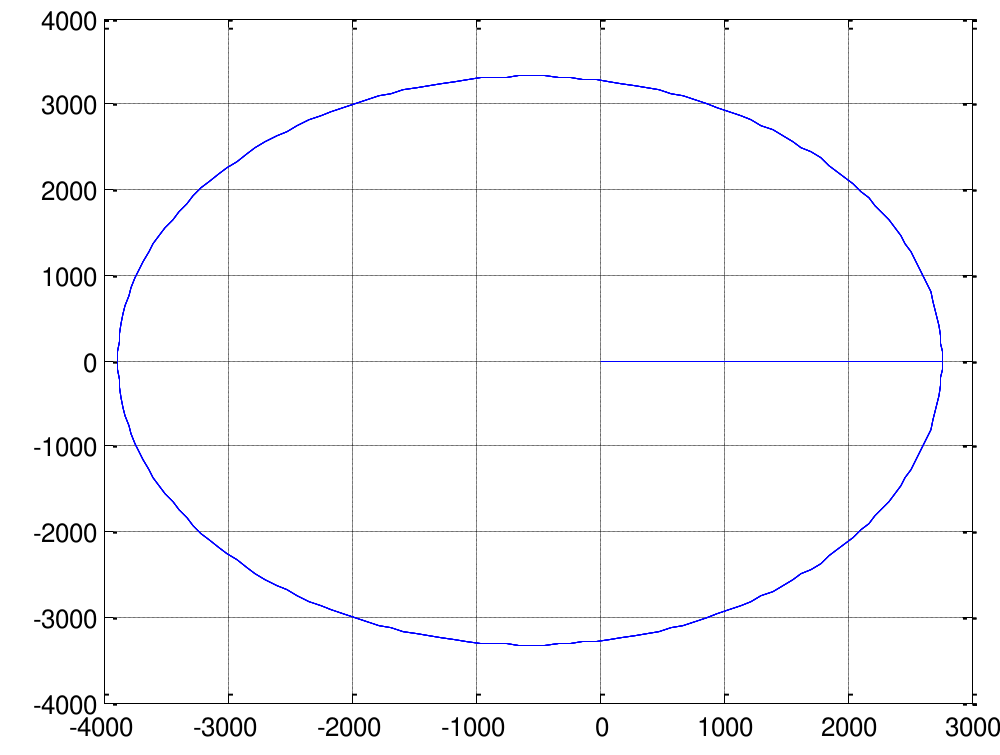# An Optimized Half-Step Scheme Third derivative Methods for Testing Higher Order Initial Value Problems

## Authors

• D. Raymond Department of Mathematics and Statistics, Federal University Wukari-Nigeria
• T. P. Pantuvo Department of Mathematics and Statistics, Federal University Wukari-Nigeria
• A. Lydia Department of Mathematics and Statistics, Federal University Wukari-Nigeria
• J. Sabo Department of Mathematics, Adamawa State University, Mubi-Nigeria
• R. Ajia Department of Mathematics and Statistics, College of Agriculture, Science and Technology, Jalingo.

## Keywords:

Half-step, Optimize Hybrid Block, Local Truncation error, Third Order Problem

## Abstract

An optimized half-step third derivative block scheme on testing third order initial value problems is presented in this article. This scheme suggests some certain points of evaluation which properly optimizes the truncation errors at point of formulas, the conditions that guarantee the properties of the method was considered and satisfied. However the develop scheme is used to test some third order optimized problems and the mathematical outcomes achieved confirms better calculation than the previous method we related with.

Dimensions

G. Dahlguist, “A special stability problem for linear multistep methods”, BIT 3 (1963) 27.

J. Kuboye, O. R. Elusakin & O. F. Quadri, “Numerical algorithm for direct solution of fourth order ordinary differential equations”, Journal of Nigerian Society of Physical Sciences 2 (2020) 218.

D. Raymond, T. Y. Kyagya, J. Sabo & A. Lydia, “numerical application of higher order linear block scheme on testing some modeled problems of fourth order problem”, African Scientific Reports 2 (2023) 2. doi:10.46481/asr.2022.2.1.67.

A. O. Adesanya, M. K. Fasansi & M. R. Odekunle, “One step three hybrid block predictor-corrector method for the solution of Applied and computational Mathematics 2 (2013) 137, doi:10.4172/21689679.1000137

O. A. Adebayo & E. O. Adebola, “one step hybrid method for the numerical solution of general third order ordinary differential equations”, International Journal of Mathematical Sciences 2 (2016) 10.

L. O. Adoghe & E. O. Omole, “A fifth-fourth continuous block implicit hybrid method for the solution of third order initial value problems in ordinary differential equations”, Applied and Computational Mathematics 8 (2019) 52.

R. Higinio, Z. Kalogiratou, T. H. Monovasilis & T. E. Simos, “An optimized two-step hybrid block method for solving general second order initial-vlue problems”, Numer. Algorithms 72 (2016) 1090.

S. H. Bothayna & A. Sadeemr, “Optimization of two-step block method with three hybrid points for solving third order initial value problems”, Journal of Nonlinear Sciences and Applications 12 (2019) 467.

S. Joshua, “Optimized two-step second derivative methods for the solutions of stiff systems”, Int. Journal of Physics Communications 6 (2022) 055016.2023-04-29

## How to Cite

Raymond, D., Pantuvo, T. P., Lydia, A., Sabo, J., & Ajia, R. (2023). An Optimized Half-Step Scheme Third derivative Methods for Testing Higher Order Initial Value Problems. African Scientific Reports, 2(1), 76. https://doi.org/10.46481/asr.2023.2.1.76

## Section

Original Research